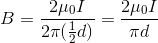# AP Physics C Electricity : Understanding Magnetic Fields and Wires

## Example Questions

### Example Question #6 : Magnetism

Two infinitely long wires having currents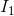and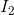are separated by a distance.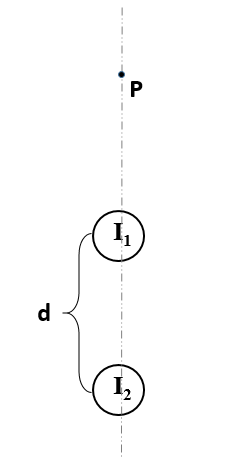The currentis 6A into the page. The currentis 9A into the page. The distance of separation is 1.5mm. The pointlies 1.5mm away fromon a line connecting the centers of the two wires.

What is the magnitude and direction of the net magnetic field at the point?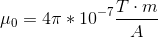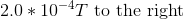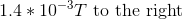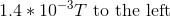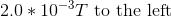Explanation:

At point, the magnetic field due topoints right (via the right hand rule) with a magnitude given by: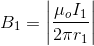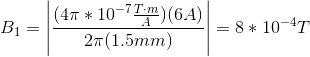At point, the magnetic field due topoints right (via the right hand rule) with a magnitude given by: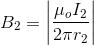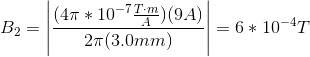The addition of these two vectors, both pointing in the same direction, results in a net magnetic field vector of magnitude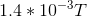to the right.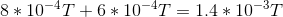### Example Question #7 : Magnetism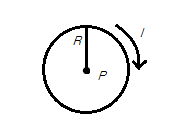Consider a current-carrying loop with current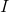, radius, and centerWhat is the direction of the magnetic field produced?

Counterclockwise

Into the screen

Clockwise

Out of the screen

There is no resultant magnetic field

Into the screen

Explanation:

The correct answer is into the page. As the current is moving clockwise, we can use our right hand rule for magnetic fields produced by a current-carrying loop. Curl the fingers of your right hand in the direction of the current. This should result in your thumb pointing toward the screen, indicating the direction of the magnetic field.

### Example Question #8 : MagnetismConsider a current-carrying loop with current, radius, and centerWhat would happen to the magnetic field at pointif the radius was halved and current was multiplied by four?

The new magnetic field would be eight times as strong as the original

The new magnetic field would be four times weaker than the original

The new magnetic field would be eight times weaker than the original

The new magnetic field would be four times as strong as the original

The new magnetic field would reverse direction

The new magnetic field would be eight times as strong as the original

Explanation:

The current flowing clockwise through the wire will induce a magentic field directed into the screen. The magnitude of such a magnetic field is given by the equation: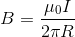Using out altered values, we can derive a ratio to determine the change in magnetic field.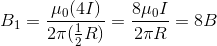The resulting field will be eight times stronger than the original.

### Example Question #9 : MagnetismConsider a current-carrying loop with current, radius, and centerWhat is the magnitude of the magnetic field at point?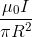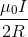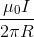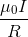There is no magnetic field at pointExplanation:

The current flowing clockwise through the wire will induce a magentic field directed into the screen. The magnitude of such a magnetic field is given by the equation:### Example Question #10 : Magnetism

Consider two long, straight, current-carrying wires at distancefrom each other, each with a current of magnitudegoing in opposite directions.

What is the magnitude of the magnetic field at a point equidistant from both wires?

Zero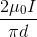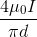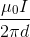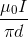Explanation:

Using our right hand rule for magnetic fields produced by current-carrying wires, we know that the magnetic field produced by each wire is in the same direction within the distance between the wires. Therefore, we know that the total magnetic field is simply the magnetic field of one of the wires multiplied by two.

Use the equation for magnetic field:Multiply by two, since the magnetic field will be equal for each wire, and substitute the given variables: